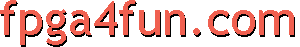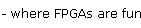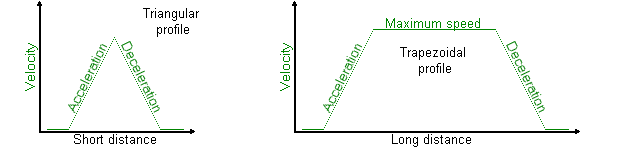## CNC 7 - Movement

##### Axes parameters

Each axis has two parameters:

• A maximum acceleration
• A maximum speed

For example, one axis could have a maximum acceleration of 20mm/s², and a maximum speed of 50mm/s.
From the motion formulas in continuous time, we can deduct than, starting from idle and using the maximum acceleration, we would reach the maximum speed after 2.5 seconds, and reach a position at that time of 62.5mm. Then using the maximum deceleration (-20mm/s²), we would move another 62.5mm, for a total of 125mm.

##### Linear motion on a single axis

Let's say we want to move one axis for a determined distance while leaving the other axes alone.

There are two cases: a short or long distance.In the first case (short distance), we are not limited by the maximum speed, while in the second (long distance), we are.
For example, assuming an acceleration of 20mm/s² and a maximum speed of 50mm/s, a distance below 125mm fits the first case, and above 125mm the second case.

##### Linear motion on multiple axes

Here we want to move in a diagonal line - multiple axes are involved and need to move simultaneously and synchronously.

Usually only one axis runs at maximum speed, and the others run slower. So you determine the "master axis" (the one that runs at maximum speed), and if a speed plateau is required (if any axis reaches its maximum speed). To move in a straight line, make sure all the axes move using the same profile.

##### Non-linear motion

Here you usually build a list of coordinates that your CNC needs to follow, including the velocities required at each coordinates. Then you calculate the accelerations required. We'll let that to you.